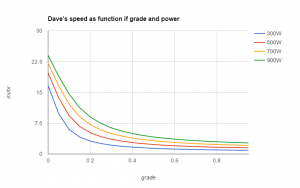# Nerding while sweating

I was slowly cranking my way of Claremont Avenue the other day on my trusty Bianchi when I started wondering why I was so slow. Well, that was easy. I’m pretty heavy and I’m somewhat out of shape. But which is more important, which would have a bigger impact if improved?

First, I used a website like this one to determine the average grade over a certain familiar portion of the route. In this case, it was 13.3%. I also have a speedometer on my bike that tells that I average about 5 mph over that stretch. Finally, I weigh about 100 kg, and my bike is another 10 kg.

So, given that the energy to raise a mass up h height is m*g*h, the power to raise a mass at r rate is m*g*r.

Result:

claremont_power: 317.67222 (watts)

That is, that’s how much power it takes to lift my mass up a hill at that rate. Note the trig to change my speed up the hill to a vertical speed. There are losses in pedaling a bike, and on the tires on the road, etc, but this is a good estimate of the overall order of how much power I can comfortably sustain. Let’s call it 300W.

Now, another thing I’ve noticed while riding is that on flat ground, I can maintain about 17 mph. In that case, I’m not adding power to climb a hill at all, all of my power is overcoming road friction and drag.

It happens that power going to aerodynamic drag goes by the cube of the velocity. (There is more going on here than wind drag, but, eh, it probably dominates at higher speeds…) So, if we assume that on level ground I’m capable of the same ~300W that I do while climbing, I can calculate the constant in:

P = c * v^3

This is a simplification of the more general equation linked above, assuming constant air density, yadda. For 17 mph and 317 W, I get about 0.72376 kg / m. kg/m is a strange dimension, but it it what it is.

So then, I wondered, how fast should I be able to go with a given power budget while climbing different grades?

I created this equation which combines the power to climb and the power to overcome drag

P = c v^3 + m g v sin(theta)

where P  is power, c  is the drag power constant calculated above, m  is mass, g  is the acceleration of gravity, and theta is the angle of the hill. (The angle is the arctangent of the grade, by the way.) Oh, and v  is my speed.

It turns out that my brain doesn’t perform the way it once did and I can’t solve that cubic equation on my own, so I resorted to a Python-based solver which is part of the sympy package.

This function gets the job done:

Note this equation has three solutions, two of which are complex. Only interested in the “real” solution.

Now, this is finally where the fun starts. Want to know how fast I can climb different grades, or how actual athletes who can summon more power than me can get up?How fast I might get up hills if I could make more power. (mass = 110 kg)

Like I said, I can make about 300W, but I saw a youtube video of a dude who could make about 1kW, at least for long enough to make toast.

Then I was wonder, would losing weight help much? It does. Interestingly, it helps on the middle grades. On the highest grades, I’m nearly stopped, and the numbers get small. On flat grades, drag (a function of my shape, not my size) dominates. But in the middle, yeah, there’s an effect.

1.Jonathan Marshall says: